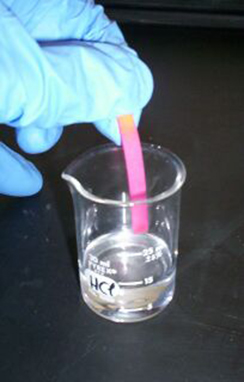# 6.5 Logarithmic properties

 Page 1 / 10
In this section, you will:
• Use the product rule for logarithms.
• Use the quotient rule for logarithms.
• Use the power rule for logarithms.
• Expand logarithmic expressions.
• Condense logarithmic expressions.
• Use the change-of-base formula for logarithms.The pH of hydrochloric acid is tested with litmus paper. (credit: David Berardan)

In chemistry, pH is used as a measure of the acidity or alkalinity of a substance. The pH scale runs from 0 to 14. Substances with a pH less than 7 are considered acidic, and substances with a pH greater than 7 are said to be alkaline. Our bodies, for instance, must maintain a pH close to 7.35 in order for enzymes to work properly. To get a feel for what is acidic and what is alkaline, consider the following pH levels of some common substances:

• Battery acid: 0.8
• Stomach acid: 2.7
• Orange juice: 3.3
• Pure water: 7 (at 25° C)
• Human blood: 7.35
• Fresh coconut: 7.8
• Sodium hydroxide (lye): 14

To determine whether a solution is acidic or alkaline, we find its pH, which is a measure of the number of active positive hydrogen ions in the solution. The pH is defined by the following formula, where $\text{\hspace{0.17em}}a\text{\hspace{0.17em}}$ is the concentration of hydrogen ion in the solution

The equivalence of $\text{\hspace{0.17em}}-\mathrm{log}\left(\left[{H}^{+}\right]\right)\text{\hspace{0.17em}}$ and $\text{\hspace{0.17em}}\mathrm{log}\left(\frac{1}{\left[{H}^{+}\right]}\right)\text{\hspace{0.17em}}$ is one of the logarithm properties we will examine in this section.

## Using the product rule for logarithms

Recall that the logarithmic and exponential functions “undo” each other. This means that logarithms have similar properties to exponents. Some important properties of logarithms are given here. First, the following properties are easy to prove.

$\begin{array}{l}{\mathrm{log}}_{b}1=0\\ {\mathrm{log}}_{b}b=1\end{array}$

For example, $\text{\hspace{0.17em}}{\mathrm{log}}_{5}1=0\text{\hspace{0.17em}}$ since $\text{\hspace{0.17em}}{5}^{0}=1.\text{\hspace{0.17em}}$ And $\text{\hspace{0.17em}}{\mathrm{log}}_{5}5=1\text{\hspace{0.17em}}$ since $\text{\hspace{0.17em}}{5}^{1}=5.$

Next, we have the inverse property.

For example, to evaluate $\text{\hspace{0.17em}}\mathrm{log}\left(100\right),$ we can rewrite the logarithm as $\text{\hspace{0.17em}}{\mathrm{log}}_{10}\left({10}^{2}\right),$ and then apply the inverse property $\text{\hspace{0.17em}}{\mathrm{log}}_{b}\left({b}^{x}\right)=x\text{\hspace{0.17em}}$ to get $\text{\hspace{0.17em}}{\mathrm{log}}_{10}\left({10}^{2}\right)=2.$

To evaluate $\text{\hspace{0.17em}}{e}^{\mathrm{ln}\left(7\right)},$ we can rewrite the logarithm as $\text{\hspace{0.17em}}{e}^{{\mathrm{log}}_{e}7},$ and then apply the inverse property $\text{\hspace{0.17em}}{b}^{{\mathrm{log}}_{b}x}=x\text{\hspace{0.17em}}$ to get $\text{\hspace{0.17em}}{e}^{{\mathrm{log}}_{e}7}=7.$

Finally, we have the one-to-one property.

We can use the one-to-one property to solve the equation $\text{\hspace{0.17em}}{\mathrm{log}}_{3}\left(3x\right)={\mathrm{log}}_{3}\left(2x+5\right)\text{\hspace{0.17em}}$ for $\text{\hspace{0.17em}}x.\text{\hspace{0.17em}}$ Since the bases are the same, we can apply the one-to-one property by setting the arguments equal and solving for $\text{\hspace{0.17em}}x:$

But what about the equation $\text{\hspace{0.17em}}{\mathrm{log}}_{3}\left(3x\right)+{\mathrm{log}}_{3}\left(2x+5\right)=2?\text{\hspace{0.17em}}$ The one-to-one property does not help us in this instance. Before we can solve an equation like this, we need a method for combining terms on the left side of the equation.

Recall that we use the product rule of exponents to combine the product of exponents by adding: $\text{\hspace{0.17em}}{x}^{a}{x}^{b}={x}^{a+b}.\text{\hspace{0.17em}}$ We have a similar property for logarithms, called the product rule for logarithms , which says that the logarithm of a product is equal to a sum of logarithms. Because logs are exponents, and we multiply like bases, we can add the exponents. We will use the inverse property to derive the product rule below.

Given any real number $\text{\hspace{0.17em}}x\text{\hspace{0.17em}}$ and positive real numbers and $\text{\hspace{0.17em}}b,$ where $\text{\hspace{0.17em}}b\ne 1,$ we will show

what are odd numbers
numbers that leave a remainder when divided by 2
Thorben
1,3,5,7,... 99,...867
Thorben
the third and the seventh terms of a G.P are 81 and 16, find the first and fifth terms.
if a=3, b =4 and c=5 find the six trigonometric value sin
pls how do I factorize x⁴+x³-7x²-x+6=0
in a function the input value is called
how do I test for values on the number line
if a=4 b=4 then a+b=
a+b+2ab
Kin
commulative principle
a+b= 4+4=8
Mimi
If a=4 and b=4 then we add the value of a and b i.e a+b=4+4=8.
Tariq
what are examples of natural number
an equation for the line that goes through the point (-1,12) and has a slope of 2,3
3y=-9x+25
Ishaq
show that the set of natural numberdoes not from agroup with addition or multiplication butit forms aseni group with respect toaaddition as well as multiplication
x^20+x^15+x^10+x^5/x^2+1
evaluate each algebraic expression. 2x+×_2 if ×=5
if the ratio of the root of ax+bx+c =0, show that (m+1)^2 ac =b^2m
By the definition, is such that 0!=1.why?
(1+cosA+IsinA)(1+cosB+isinB)/(cos@+isin@)(cos$+isin$)
hatdog
Mark
jaks
Ryan

#### Get Jobilize Job Search Mobile App in your pocket Now!ByBy OpenStaxBy Nick SwainBy Michael SagBy Stephen VoronBy Sarah WarrenBy OpenStaxBy JavaChamp TeamBy Saylor FoundationBy Brooke DelaneyBy OpenStax